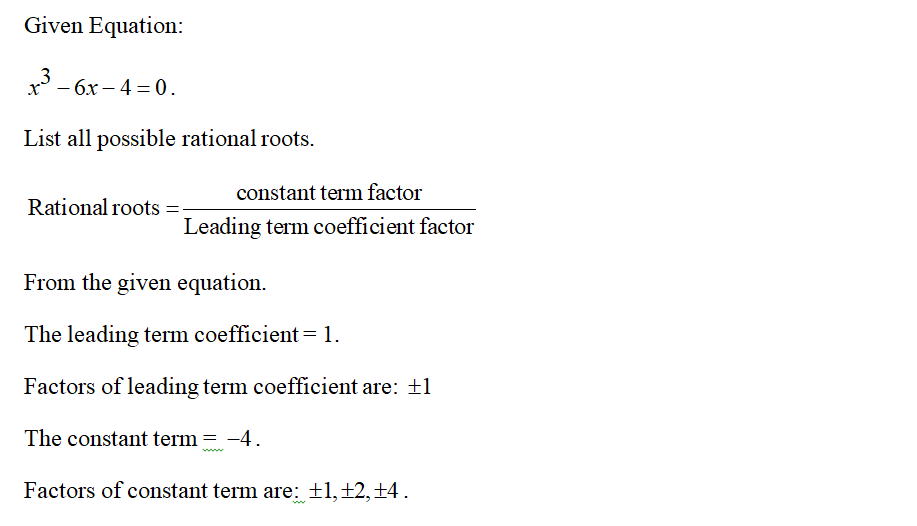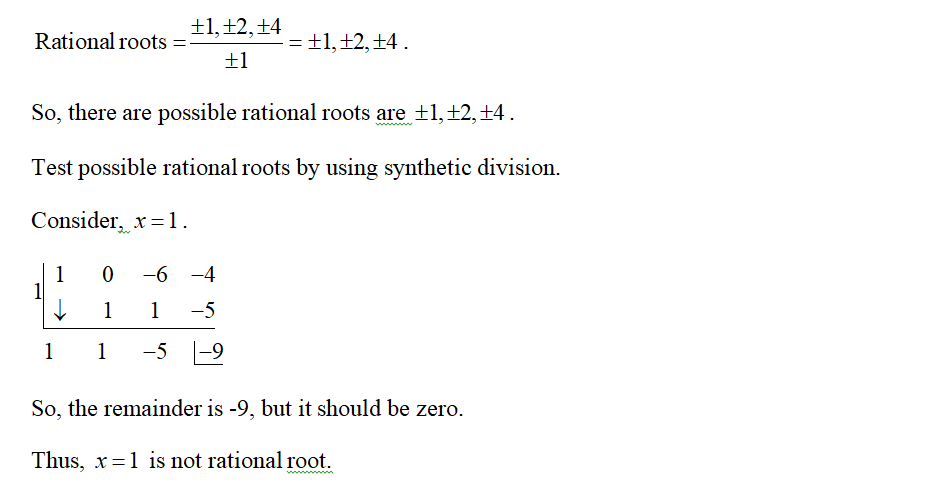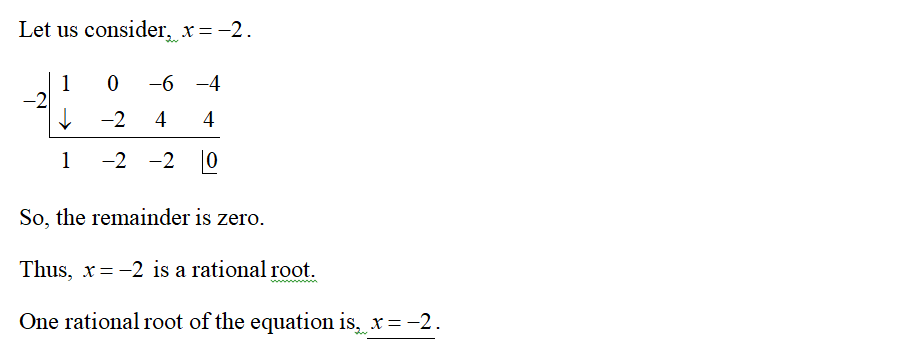# 1.answer the following questions about the equation below x^3-6x-4=0 -One rational of the given equarion is ___- the solution set is {____}

Question
1 views

1.

x^3-6x-4=0

-One rational of the given equarion is ___

- the solution set is {____}

check_circle

Step 1Step 2Step 3...

### Want to see the full answer?

See Solution

#### Want to see this answer and more?

Solutions are written by subject experts who are available 24/7. Questions are typically answered within 1 hour.*

See Solution
*Response times may vary by subject and question.
Tagged in